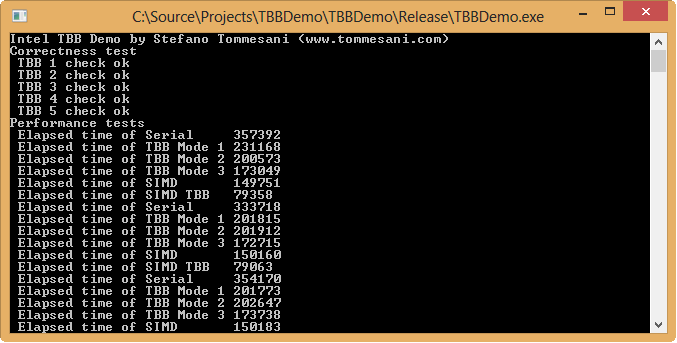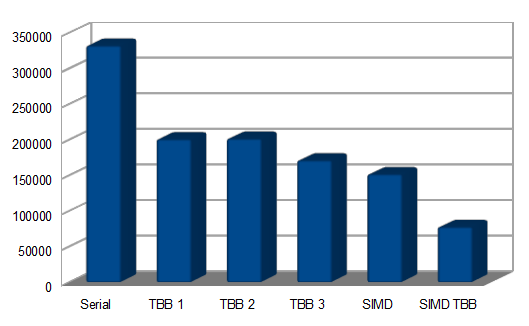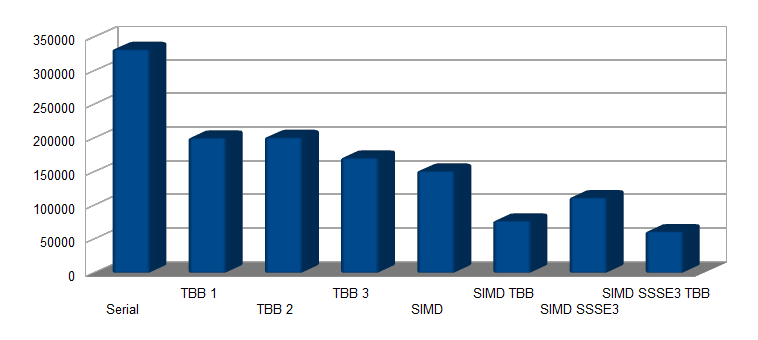This is not the first article on this site that discusses how to use the Intel Thread Building Blocks library to spread the computation of an image-processing kernel over multiple threads: the article named “Multi thread loops with Intel TBB” showed how to do it with Intel TBB 2.x. However, due to the enhancements of Intel TBB 4.x, and the availability of C++ 11 compliant compilers such as Microsoft Visual C++ 2012, it is now possible to write more compact and easy to understand code than before.The starting point is a scalar C function that converts a RGB or RGBA image into a grayscale, luma-only image:

``// ProcessRGBSerial // reference serial code that converts a given RGB image to a luma-only image // both input and output image are unidimensional arrays of ImageWidth * ImageHeight pixels // PixelOffset is the distance in bytes between two pixels in the input RGB image (RGB24 -> PixelOffset = 3, RGBA32 -> PixelOffset = 4)  void ProcessRGBSerial(void *SourceImage, void *YImage, int ImageWidth, int ImageHeight, int PixelOffset) {     unsigned char *SourceImagePtr = (unsigned char *)SourceImage;     unsigned char *YImagePtr = (unsigned char *)YImage;      for (int i = (ImageWidth * ImageHeight); i > 0; i--)     {         int YValue = (SourceImagePtr * Y_RED_SCALE  ) +                      (SourceImagePtr * Y_GREEN_SCALE) +                      (SourceImagePtr * Y_BLUE_SCALE );         SourceImagePtr += PixelOffset;         YValue += 1 << (SCALING_LOG - 1);         YValue >>= SCALING_LOG;         if (YValue > 255)             YValue = 255;         *YImagePtr = (unsigned char)YValue;         YImagePtr++;     } } ``

Please refer to the full source code at the bottom of the page for the declaration of constants, or just download the whole project and open it in Microsoft Visual C++ 2012.

Before starting to rewrite this function to exploit multiple threads, we add the needed header files of Intel TBB:

``#include <task_scheduler_init.h> using namespace tbb; #include <parallel_for.h> #include <blocked_range.h> #include <blocked_range2d.h> ``

Now we are ready to rewrite the ProcessRGBSerial function to use TBB’s threads. In the first step, we will use the same method of TBB 2.x:

``// ProcessRGBTBB1 // first version of multi-threaded code using Intel TBB // it is necessary to define a struct (RGBToGrayConverter) with a () operator that hosts the kernel of the computation // all the parameters necessary to the computation are copied inside the struct  struct RGBToGrayConverter {     unsigned char *SourceImagePtr;     unsigned char *YImagePtr;     int PixelOffset;     void operator( )( const blocked_range<int>& range ) const {         unsigned char *LocalSourceImagePtr = SourceImagePtr;         unsigned char *LocalYImagePtr = YImagePtr;         LocalSourceImagePtr += range.begin() * PixelOffset;         LocalYImagePtr += range.begin();         for( int i=range.begin(); i!=range.end( ); ++i )         {             int YValue = (LocalSourceImagePtr * Y_RED_SCALE  ) +                          (LocalSourceImagePtr * Y_GREEN_SCALE) +                          (LocalSourceImagePtr * Y_BLUE_SCALE );             LocalSourceImagePtr += PixelOffset;             YValue += 1 << (SCALING_LOG - 1);             YValue >>= SCALING_LOG;             if (YValue > 255)                 YValue = 255;             *LocalYImagePtr = (unsigned char)YValue;             LocalYImagePtr++;         }     } };  void ProcessRGBTBB1(void *SourceImage, void *YImage, int ImageWidth, int ImageHeight, int PixelOffset) {     RGBToGrayConverter RGBToGrayConverterPtr;     RGBToGrayConverterPtr.PixelOffset = PixelOffset;     RGBToGrayConverterPtr.SourceImagePtr = (unsigned char *)SourceImage;     RGBToGrayConverterPtr.YImagePtr = (unsigned char *)YImage;      parallel_for( blocked_range<int>( 0, (ImageWidth * ImageHeight)), RGBToGrayConverterPtr); } ``

The computational kernel is contained in the () operator of the RGBToGrayConverter struct. All the I/O parameters of the computation kernel must be added to the struct, and they must be initialized by the calling ProcessTGBTBB1 function before starting the processing via parallel_for. Each time the operator () of the struct is called, the thread gets a sub-range of the whole image to work on, contained in the blocked_range parameter, so it computes the pixels between range.begin() and range.end(). Even if there is a significant amount of boiler-plate code around it, the core of the kernel is mostly unchanged, so most of the source serial code is retained.

Still, we can do better than this. By moving the image-processing kernel in a lambda defined inside the call to parallel_for, we can completely avoid the declaration of a new struct:

``// ProcessRGBTBB2 // by using a lambda that copies the required information automatically [=], the kernel of the computation is contained // inside the invocation to parallel_for  void ProcessRGBTBB2(void *SourceImage, void *YImage, int ImageWidth, int ImageHeight, int PixelOffset) {     parallel_for( blocked_range<int>( 0, (ImageWidth * ImageHeight)),         [=](const blocked_range<int>& r) {             unsigned char *LocalSourceImagePtr = (unsigned char *)SourceImage;             unsigned char *LocalYImagePtr = (unsigned char *)YImage;             LocalSourceImagePtr += r.begin() * PixelOffset;             LocalYImagePtr += r.begin();              for(size_t i = r.begin(); i != r.end(); ++i)             {                 int YValue = (LocalSourceImagePtr * Y_RED_SCALE  ) +                              (LocalSourceImagePtr * Y_GREEN_SCALE) +                              (LocalSourceImagePtr * Y_BLUE_SCALE );                 LocalSourceImagePtr += PixelOffset;                 YValue += 1 << (SCALING_LOG - 1);                 YValue >>= SCALING_LOG;                 if (YValue > 255)                     YValue = 255;                 *LocalYImagePtr = (unsigned char)YValue;                 LocalYImagePtr++;             }           }         ); } ``

While the first parameter to the parallel_for invocation is still a blocked_range defined as before, the second parameter is now the declaration of a lambda, so that the image processing kernel is inside the calling function. This parallel code is remarkably similar to the original serial code, the major difference being that processing is applied to a slice of the input image instead of the whole image, as shown in the blocked_range parameter. Please note that [=] in the declaration of the lambda automatically copies the required I/O parameters used by the lambda, so we do not have to add these variables to a struct and fill them one by one, a boring and error-prone task.

Since the images were defined as one-dimensional arrays, using a 1D blocked_range to work on a slice of the image was a natural match. Still, it is possible to use a 2D blocked_range, unsurprinsingly named blocked_range2d, to work on 2D tiles instead of 1D slices:

``// ProcessRGBTBB3 // even if working with images expressed as unidimensional array does not require it, a 2D range instead of a 1D one // works on a tile of the given images at a time // the location and dimensions of the tile are contained in the rows and cols of the blocked_range2d  void ProcessRGBTBB3(void *SourceImage, void *YImage, int ImageWidth, int ImageHeight, int PixelOffset) {     parallel_for( blocked_range2d<int,int>( 0, ImageHeight, 0, ImageWidth),         [=](const blocked_range2d<int,int>& r) {             int StartX = r.cols().begin();             int StopX = r.cols().end();             int StartY = r.rows().begin();             int StopY = r.rows().end();              for (int y = StartY; y != StopY; y++)             {                 unsigned char *LocalSourceImagePtr = (unsigned char *)SourceImage + (StartX + y * ImageWidth) * PixelOffset;                 unsigned char *LocalYImagePtr = (unsigned char *)YImage + (StartX + y * ImageWidth);                 for (int x = StartX; x != StopX; x++)                 {                     int YValue = (LocalSourceImagePtr * Y_RED_SCALE ) +                              (LocalSourceImagePtr * Y_GREEN_SCALE) +                              (LocalSourceImagePtr * Y_BLUE_SCALE );                     LocalSourceImagePtr += PixelOffset;                     YValue += 1 << (SCALING_LOG - 1);                     YValue >>= SCALING_LOG;                     if (YValue > 255)                         YValue = 255;                     *LocalYImagePtr = (unsigned char)YValue;                     LocalYImagePtr++;                 }             }           }         ); } ``

This time we have a 2D range, and we get the interval in both the horizontal and vertical direction by reading the rows() and cols() of the given blocked_range2d.

Still, we can do better than this, by rewriting the kernel using SIMD instructions (in this case, we will use SSE2 instructions). To keep the code simple and readable, we will assume that ImageWidth * ImageHeight is an integer multiple of 4 (for a generic dimension, we should add a serial loop at the end that computes the missing bytes in the output image). As the input buffer is not guaranteed to be aligned on 16-bytes boundaries, the loads from the input image are unaligned using the _mm_loadu_si128() intrinsics. I have added the corresponding serial C code lines inside the SIMD loop, so that you can match the behaviour of SSE2 instructions to the reference C code, as the SIMD code bears little if any resemblance to the C code. Please note that the SIMD code is not a direct replacement for the serial C code, as each iteration of this loop produces 4 output pixels, while the serial C code produces only 1 output pixel per loop.

``// ProcessRGBSIMD // SSE2 version of Serial code // both input and output image are unidimensional arrays of ImageWidth * ImageHeight pixels // assumes that PixelOffset is 4 (RGBA image) and that ImageWidth * ImageHeight * PixelOffset is a multiple of 16 // does not assume that the input image is aligned on 16 bytes  void ProcessRGBSIMD(void *SourceImage, void *YImage, int ImageWidth, int ImageHeight, int PixelOffset) {     __m128i RGBScale = _mm_set_epi16(0, Y_BLUE_SCALE, Y_GREEN_SCALE, Y_RED_SCALE, 0, Y_BLUE_SCALE, Y_GREEN_SCALE, Y_RED_SCALE);     __m128i ShiftScalingAdjust = _mm_set1_epi32(1 << (SCALING_LOG - 1));     __m128i *SourceImagePtr = (__m128i *)SourceImage;     int *YImagePtr = (int *)YImage;      for (int i = (ImageWidth * ImageHeight); i > 0; i -= 4)  // 4 pixels per loop     {         __m128i RGBValue = _mm_loadu_si128(SourceImagePtr);         SourceImagePtr++;         __m128i LowRGBValue = _mm_unpacklo_epi8(RGBValue, _mm_setzero_si128());         __m128i HighRGBValue = _mm_unpackhi_epi8(RGBValue, _mm_setzero_si128());         // int YValue = (SourceImagePtr * Y_RED_SCALE  ) +         //             (SourceImagePtr * Y_GREEN_SCALE) +         //             (SourceImagePtr * Y_BLUE_SCALE );         __m128i LowYValue = _mm_madd_epi16(LowRGBValue, RGBScale);         __m128i HighYValue = _mm_madd_epi16(HighRGBValue, RGBScale);         LowYValue = _mm_add_epi32(LowYValue, _mm_slli_epi64(LowYValue, 32));         HighYValue = _mm_add_epi32(HighYValue, _mm_slli_epi64(HighYValue, 32));         // YValue += 1 << (SCALING_LOG - 1);         LowYValue = _mm_add_epi32(LowYValue, ShiftScalingAdjust);         HighYValue = _mm_add_epi32(HighYValue, ShiftScalingAdjust);         // YValue >>= SCALING_LOG;         LowYValue = _mm_srli_epi64(LowYValue, 32 + SCALING_LOG);         HighYValue = _mm_srli_epi64(HighYValue, 32 + SCALING_LOG);         __m128i YValue =  _mm_packs_epi32(LowYValue, HighYValue);         YValue =  _mm_packs_epi32(YValue, _mm_setzero_si128());         // if (YValue > 255)         //    YValue = 255;         YValue =  _mm_packus_epi16(YValue, YValue);         *YImagePtr = _mm_cvtsi128_si32(YValue);         YImagePtr++;     } } ``

The final step is merging the SIMD kernel with the TBB multi-thread approach so that we can achieve the highest level of performance:

``// ProcessRGBTBBSIMD // by using a lambda that copies the required information automatically [=], the kernel of the computation is contained // inside the invocation to parallel_for  void ProcessRGBTBBSIMD(void *SourceImage, void *YImage, int ImageWidth, int ImageHeight, int PixelOffset) {     __m128i RGBScale = _mm_set_epi16(0, Y_BLUE_SCALE, Y_GREEN_SCALE, Y_RED_SCALE, 0, Y_BLUE_SCALE, Y_GREEN_SCALE, Y_RED_SCALE);     __m128i ShiftScalingAdjust = _mm_set1_epi32(1 << (SCALING_LOG - 1));     __m128i *SourceImagePtr = (__m128i *)SourceImage;     int *YImagePtr = (int *)YImage;      parallel_for( blocked_range<int>( 0, (ImageWidth * ImageHeight)),         [=](const blocked_range<int>& r) {             unsigned char *LocalSourceImagePtr = (unsigned char *)SourceImage;             unsigned char *LocalYImagePtr = (unsigned char *)YImage;             LocalSourceImagePtr += r.begin() * PixelOffset;             LocalYImagePtr += r.begin();             __m128i *SourceImagePtr = (__m128i *)LocalSourceImagePtr;             int *YImagePtr = (int *)LocalYImagePtr;              for (size_t i = r.begin(); i != r.end(); i+=4)             {                 __m128i RGBValue = _mm_loadu_si128(SourceImagePtr);                 SourceImagePtr++;                 __m128i LowRGBValue = _mm_unpacklo_epi8(RGBValue, _mm_setzero_si128());                 __m128i HighRGBValue = _mm_unpackhi_epi8(RGBValue, _mm_setzero_si128());                 // int YValue = (SourceImagePtr * Y_RED_SCALE  ) +                 //             (SourceImagePtr * Y_GREEN_SCALE) +                 //             (SourceImagePtr * Y_BLUE_SCALE );                 __m128i LowYValue = _mm_madd_epi16(LowRGBValue, RGBScale);                 __m128i HighYValue = _mm_madd_epi16(HighRGBValue, RGBScale);                 LowYValue = _mm_add_epi32(LowYValue, _mm_slli_epi64(LowYValue, 32));                 HighYValue = _mm_add_epi32(HighYValue, _mm_slli_epi64(HighYValue, 32));                 // YValue += 1 << (SCALING_LOG - 1);                 LowYValue = _mm_add_epi32(LowYValue, ShiftScalingAdjust);                 HighYValue = _mm_add_epi32(HighYValue, ShiftScalingAdjust);                 // YValue >>= SCALING_LOG;                 LowYValue = _mm_srli_epi64(LowYValue, 32 + SCALING_LOG);                 HighYValue = _mm_srli_epi64(HighYValue, 32 + SCALING_LOG);                 __m128i YValue =  _mm_packs_epi32(LowYValue, HighYValue);                 YValue =  _mm_packs_epi32(YValue, _mm_setzero_si128());                 // if (YValue > 255)                 //    YValue = 255;                 YValue =  _mm_packus_epi16(YValue, YValue);                 *YImagePtr = _mm_cvtsi128_si32(YValue);                 YImagePtr++;             }           }         ); } ``

Now we have arrived to an high-performance solution, but code readibility has been severely reduced, mostly due to the SIMD instructions (even if intrinsics are a bit more readable than plain assembly code).

It is time to check how fast these code fragments run: the following graph shows the results on an Intel i3 2570, a 2 cores / 4 HT threads CPUThe Serial C code is obviously the slowest one, and will represent the baseline performance level. Moving to TBB multi-threaded code, both the code using lambda and struct have nearly equal performance, with a 65% speedup over serial code. Using tiling instead of a slice of the input image improves performance significantly, bringing the speed-up to 94%. The SSE2-based solution is faster even if it uses just a thread, running 119% than the baseline code. Merging multi-threads and SSE2 kernel code brings an impressive result, with a 327% speed-up!

You can download the full Microsoft Visual C++ 2012 project, named TBBDemo, in the Download area, it works in Visual C++ Express too! I really urge you to download and play with the code, as it takes a short time to become familiar with the new style based on lambdas, and it is a lot easier both to read and write.

## Update (April 29th, 2013)

Usage a specific SSSE3 instruction named PHADDD (packed horizontal add of dwords) replaces a couple of shift + add instructions, and as these instructions are on the critical path, the performance gain is noticeable, as we will see later. Still, in production-quality code, you should check that SSSE3 is supported on the current CPU, as pre-Core2 processors do not support it.

``// ProcessRGBSIMD2 // SSSE3 version of Serial code // both input and output image are unidimensional arrays of ImageWidth * ImageHeight pixels // assumes that PixelOffset is 4 (RGBA image) and that ImageWidth * ImageHeight * PixelOffset is a multiple of 16 // does not assume that the input image is aligned on 16 bytes  void ProcessRGBSIMD2(void *SourceImage, void *YImage, int ImageWidth, int ImageHeight, int PixelOffset) {     __m128i RGBScale = _mm_set_epi16(0, Y_BLUE_SCALE, Y_GREEN_SCALE, Y_RED_SCALE, 0, Y_BLUE_SCALE, Y_GREEN_SCALE, Y_RED_SCALE);     __m128i ShiftScalingAdjust = _mm_set1_epi32(1 << (SCALING_LOG - 1));     __m128i *SourceImagePtr = (__m128i *)SourceImage;     __m128i ZeroConst = _mm_setzero_si128();     int *YImagePtr = (int *)YImage;      for (int i = (ImageWidth * ImageHeight); i > 0; i -= 4)  // 4 pixels per loop     {         __m128i RGBValue = _mm_loadu_si128(SourceImagePtr);         SourceImagePtr++;         __m128i LowRGBValue = _mm_unpacklo_epi8(RGBValue, ZeroConst);         __m128i HighRGBValue = _mm_unpackhi_epi8(RGBValue, ZeroConst);         // int YValue = (SourceImagePtr * Y_RED_SCALE  ) +         //             (SourceImagePtr * Y_GREEN_SCALE) +         //             (SourceImagePtr * Y_BLUE_SCALE );         __m128i LowYValue = _mm_madd_epi16(LowRGBValue, RGBScale);         __m128i HighYValue = _mm_madd_epi16(HighRGBValue, RGBScale);         __m128i YValue = _mm_hadd_epi32(LowYValue, HighYValue);         // YValue += 1 << (SCALING_LOG - 1);         YValue = _mm_add_epi32(YValue, ShiftScalingAdjust);         // YValue >>= SCALING_LOG;         YValue = _mm_srli_epi32(YValue, SCALING_LOG);         YValue =  _mm_packs_epi32(YValue, YValue);         // if (YValue > 255)         //    YValue = 255;         YValue =  _mm_packus_epi16(YValue, YValue);         // *YImagePtr = (unsigned char)YValue;         *YImagePtr = _mm_cvtsi128_si32(YValue);         YImagePtr++;     } } ``

So, how fast does it run? While the SSE2-optimized code was 2.2 times faster than the serial code, this version using PHADDD achieves 3x speed-up, and adding TBB multi-threading brings the overall speed-up to a respectable 5.4x. A futher 10% improvement can be obtained by unrolling the loop one time, and pairing the instructions of the separate iterations, as there is low pressure on XMM registers and most instructions are on the critical path, so there is a significant number of execution slots available for other instructions.` `